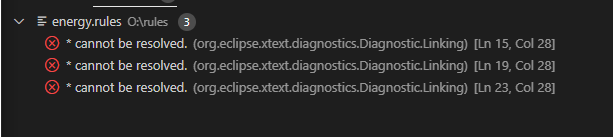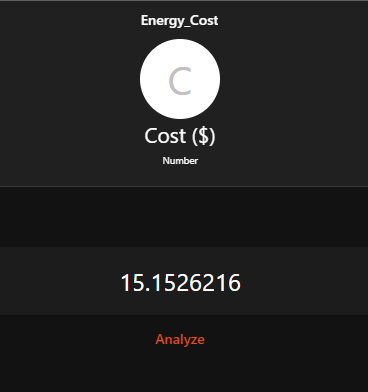# [SOLVED] Energy Calculation for Off & On peak usage

I do apologize in advance as I know there are several topics about this subject but I can’t seem to find an answer to my (fairly) trivial problem I’m having.

I have the below rule defined but am getting an error on multiplication (*) symbol saying it cannot be resolved.

The Energy_Cost is defined as a Thing:number. I have also tried it as a string but get further errors.

``````val minute = now.getHour() * 60 + now.getMinute()

rule "Energy Cost"
when
Item Energy_Watts changed
then
var cost = Energy_Watts.state instanceof Number
var Number OFFrate = 0.0074
var Number ONrate = 0.0152
var Number MIDrate = 0.0102

//Off-Peak from 7AM to 7PM
if (minute > 1140 || minute < 420) {
Energy_Cost = cost * OFFrate
}
//On-Peak from 11AM to 5PM
if (minute > 660 || minute < 1020) {
Energy_Cost = cost * ONrate
}
//Other times are MidPeak (7AM to 11AM & 5PM to 7PM)
else {
Energy_Cost = cost * MIDrate
}

end

``````

I have also tried changing the Energy_Watts.state to as Number and other ways but keep getting the error.
This variable (Energy_Watts) is also defined as a number.I know it has something to do with trying my variable types but can’t seem to figure out where I’m going wrong.

Anyone have any ideas?

Thank you kindlyCan you show us what you mean here?

Hi.

Try:

``````var cost = Energy_Watts.state as DecimalType

var OFFrate = 0.0074

Energy_Cost.state = cost * OFFrate
``````

Energy_Cost is not defined anywhere in the rule. If Energy_Cost is an item use

Energy_Cost.sendCommand(cost * ONrate)

Thank everyone for all the suggestions and replies.

@hafniumzinc

Item is defined as a number, sorry Item not Thing.I updated the code using both suggestions, switch to as DecimalType but then I was getting error about BigDecimal to NumberItems

So then as @Jogobo suggested, I edited to use sendCommand and it works now, which is why you’re seeing the number populated as 15.152 in the first screenshot.

Problem is now when I try to input this into grafana I get the
“unsupported mean iterator type *query.stringinterruptiterator” error because it sees it as a string type, even though its defined as number.

I know this wasn’t part of the original question, should this be marked a resolved and a new thread started?

Thanks again

This seems to become a grafana problem now. I don’t think this is the right place for grafana questions and qualified answers might be quite unlikely.